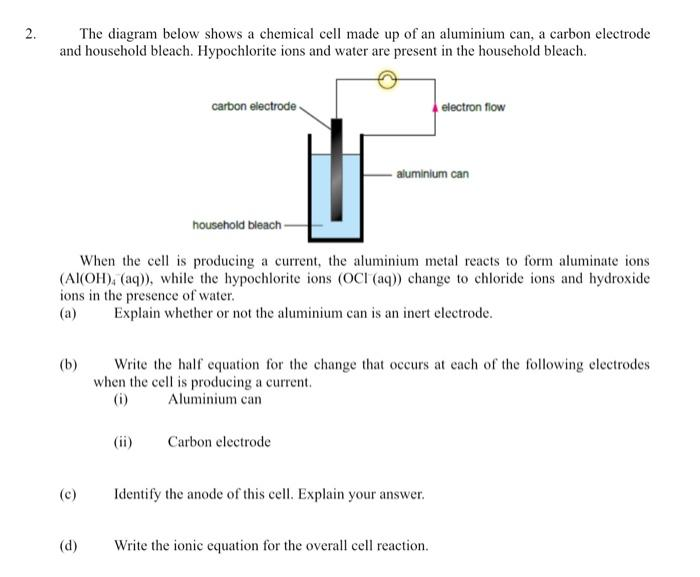# (Solved): 2. The diagram below shows a chemical cell made up of an aluminium can, a carbon electrode and house ...

2. The diagram below shows a chemical cell made up of an aluminium can, a carbon electrode and household bleach. Hypochlorite ions and water are present in the household bleach. (b) (c) (d) carbon electrode When the cell is producing a current, the aluminium metal reacts to form aluminate ions (Al(OH)4 (aq)), while the hypochlorite ions (OCI (aq)) change to chloride ions and hydroxide ions in the presence of water. (a) Explain whether or not the aluminium can is an inert electrode. household bleach (ii) aluminium can Write the half equation for the change that occurs at each of the following electrodes when the cell is producing a current. (i) Aluminium can Carbon electrode electron flow Identify the anode of this cell. Explain your answer. Write the ionic equation for the overall cell reaction.The diagram below shows a chemical cell made up of an aluminium can, a carbon electrode and household bleach. Hypochlorite ions and water are present in the household bleach. When the cell is producing a current, the aluminium metal reacts to form aluminate ions , while the hypochlorite ions change to chloride ions and hydroxide ions in the presence of water. (a) Explain whether or not the aluminium can is an inert electrode. (b) Write the half equation for the change that occurs at each of the following electrodes when the cell is producing a current. (i) Aluminium can (ii) Carbon electrode (c) Identify the anode of this cell. Explain your answer. (d) Write the ionic equation for the overall cell reaction.

We have an Answer from Expert